Courses
Courses for Kids
Free study material
Free LIVE classes
More

# Long Division Method with Regrouping and Without RemainderLIVE
Join Vedantu’s FREE Mastercalss

## Introduction to Division with Regrouping

Division is an important concept in mathematics. It can be used for division of numbers and for division of anything among our daily life use. Therefore, it is important to understand how to divide numbers and how to regroup them into different groups using a mathematical method called division with regrouping.

Division with regrouping is a very common mathematical problem. It is one of the most difficult problems to solve in the real world. The solution to this problem depends on the exact nature of the problem and its complexity. The division with regrouping problem can be solved using different methods, depending on which method you choose to solve it. The following section will explain how each method works, and also give some examples where each method has been used.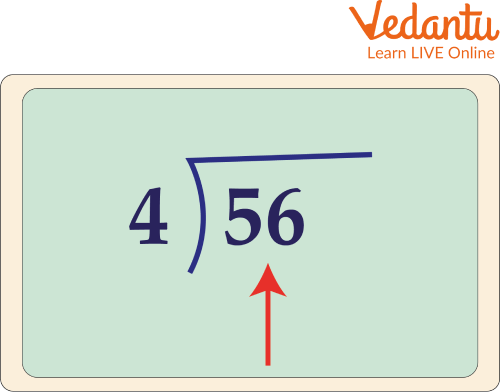Division

## Long Division Method

Long division is one of the most difficult mathematical operations. It is also a very useful technique for solving many problems in maths. In math, long division is a method for dividing large numbers into steps or parts, breaking the division problem into a sequence of easier steps. It is the most common method used to solve problems based on division.

Observe the following long division to see the divisor, the dividend, the quotient, and the remainder.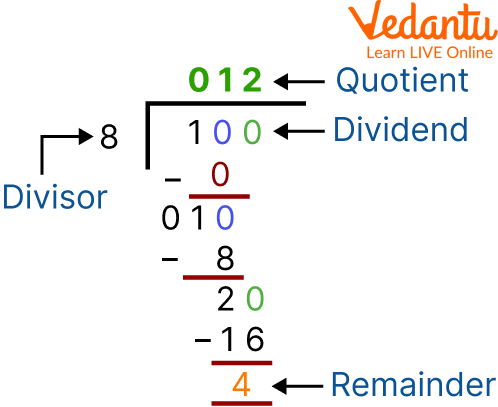Long division

## How to Solve Long Division Method with Regrouping?

Understanding a few stages where the divisor and dividend are separated by a right parenthesis or the vertical bar is necessary for using the long division method to solve problems. One must follow the stages for long division listed below in order to comprehend the procedure.

• To solve the long division method, for the first steps, the first digit of the dividend has to be taken from the left, where this digit is greater than or equal to the divisor.

• In the next step, divide by the divisor and write the above answer as a quotient.

• In this step subtract the result from the marks and write down the difference.

• In this step the next digit of the dividend has to be written down.

• After that, repeat this step.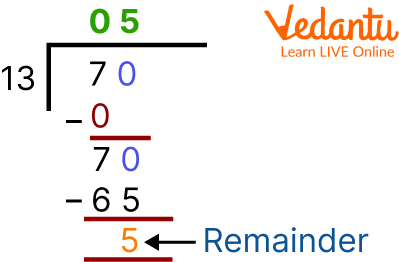Division

## Long Division Method with Regrouping and Without Remainder Example

Here are examples related to the long division method, which are as follows:

Q1. 468 ÷ 3

Ans: Let us follow the division along with the given steps.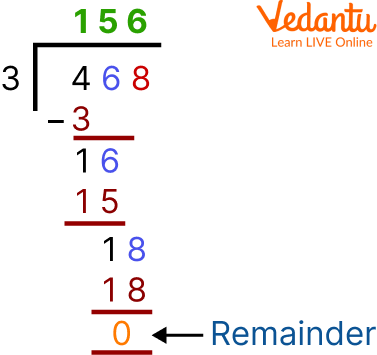Division of 468 by 3

Step I: Begin with hundreds digit

4 hundreds $\div 3=1$ hundred with remainder 1 hundred

Step II: Bring down 6 tens to the right of 1 hundred

1 hundred $+6$ tens $=16$ tens

Step III: 16 tens $\div 3=5$ tens with remainder 1 ten

Step IV: Bring down 8 ones to the right of 1 ten

1 ten $+8$ ones $=18$ ones

Step V: 18 ones $\div 3=6$ ones

Therefore, 468 ÷ 3 = 156

Q2. 9120 ÷ 5

Ans: Let us follow the division along with the given steps.

Step I: Begin with thousands digit

9 thousands $\div 5=1$ thousand with remainder 4 thousands

Step II: Bring down 1 hundred to the right of 4 thousands

Step III: Now 4 thousands $+1$ hundred $=41$ hundreds

Step IV: Now 41 hundreds $\div 5=8$ hundreds with remainder

1 hundred

Step V: Bring down 2 tens to the right of 1 hundred

Step VI: Now 1 hundred $+2$ tens $=12$ tens

Step VII: So, 12 tens $\div 5=2$ with remainder 2 tens

Step VIII: Bring down zero to the right of 2 tens

So, 2 tens $+0$ ones $=20$ ones

Now 20 ones $\div 5=4$ ones

Therefore, 9120 ÷ 5 = 1824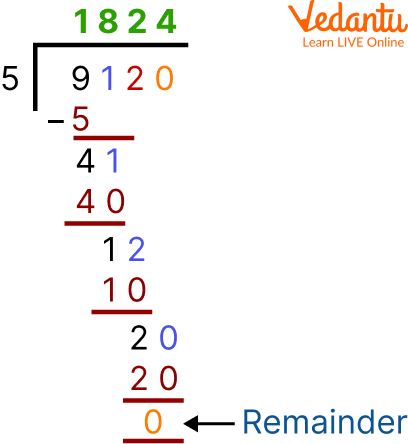Division of 9120 by 5

## Long Division Method with Regrouping and Without Remainder Worksheet

Here are worksheet related to the long division method, which are as follows;

Q1. Divide 840 by 8 using the long division method.

Ans. 105

Q2. Divide 321 by 3 using the long division method.

Ans. 107

Q3. Divide 545 by 5 using the long division method.

Ans. 109

Q4. Divide 303 by 3 using the long division method.

Ans. 101

Q5. Divide 2322 by 2 using the long division method.

Ans. 1161

## Summary

The division of a number by another number is a fundamental mathematical operation. This is often called a regrouping operation. In this section, we explored the concept of regrouping in mathematics and how it can be applied to Division. Division with regrouping is a method of dividing a number by two and getting a remainder. It is one of the most important number division operations in mathematics.

The division with regrouping is one of the most important number division operations in mathematical analysis. In fact, it is often used to simplify problems that involve fractions. The method works well when there are only two numbers involved and can be easily applied to real-world problems involving fractions as well.

Last updated date: 25th Sep 2023
Total views: 77.7k
Views today: 0.77k

## FAQs on Long Division Method with Regrouping and Without Remainder

1. What does division mean by regrouping?

When performing operations like addition and subtraction on two-digit integers or bigger, regrouping is the process of forming groups of tens. Regrouping refers to the process of performing an operation by rearranging groups in place of value.

2. What is the long division of polynomials?

In algebra, the long division of polynomials is an algorithm to divide a polynomial by another polynomial of the same or the lower degree. For example, $\left(4 x^2-5 x-21\right)$ is a polynomial that can be divided by $(x-3)$ following some defined rules, which will result in $4 x+7$ as the quotient.

3. What division methods are there?

According to the degree of difficulty, there are three different sorts of division procedures. These include the bus stop method, extended division method, and the chunking approach, often known as division by repeated subtraction.